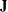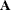VARNAMES Statement

VARNAMES name-assignment <, name-assignment …> ;

VARNAME name-assignment <, name-assignment …> ;

VNAMES name-assignment <, name-assignment …> ;

where name-assignment represents one of the following forms: matrix-name variable-names matrix-name = [variable-names] matrix-name = matrix-name

You can use the VARNAMES statement in connection with the COSAN modeling language to assign variable names for matrices. The matrix-name refers to any matrix you define in the COSAN statement. The variable-names that follow the matrix-name are assigned to the column variables of the matrix of interest. This applies to the first two types of VARNAMES specifications. For example,

varnames  F   f1-f3;

is exactly the same as

varnames  F = [ f1-f3 ];

Both of these assign f1, f2, and f3 as the names for the first three column variables of matrix F.

You can also use another kind of name-assignment in connection with a COSAN statement. Two matrix names equated by an equal sign assign the column names of the matrix on the right-hand side to the column names of the matrix on the left-hand side. This assignment assumes that the column names of at least one of the two matrices are already defined. For example, assuming thatandare model matrices defined in a COSAN statement, the following VARNAMES statement specification specifies that bothandhave the same set of column variable names V1V6 and F1F3:

varnames  J = [ V1-V6 F1-F3 ] ,
A = J ;

This is the same as the following specification:

varnames  J = [ V1-V6 F1-F3 ] ,
A = [ V1-V6 F1-F3 ] ;

The VARNAMES statement appears to enable you to specify only the column variable names for matrices. However, PROC CALIS also uses these column variable names to assign row variable names of the related matrices in the covariance and mean structure formulas for the COSAN model. PROC CALIS uses the following rules to determine the row variable names of a matrix in the model:

• If a matrix is the first matrix of any term in the covariance or mean structure formula, the row variable names are the names of the manifest variables.

• If a matrix is the central covariance matrix of any term in the covariance structure formula, the row variable names are the same as the column variable names.

• For any other matrices, the row variable names are the same as the column variable names of the preceding matrix in the multiplicative formula for the covariance or mean structures.